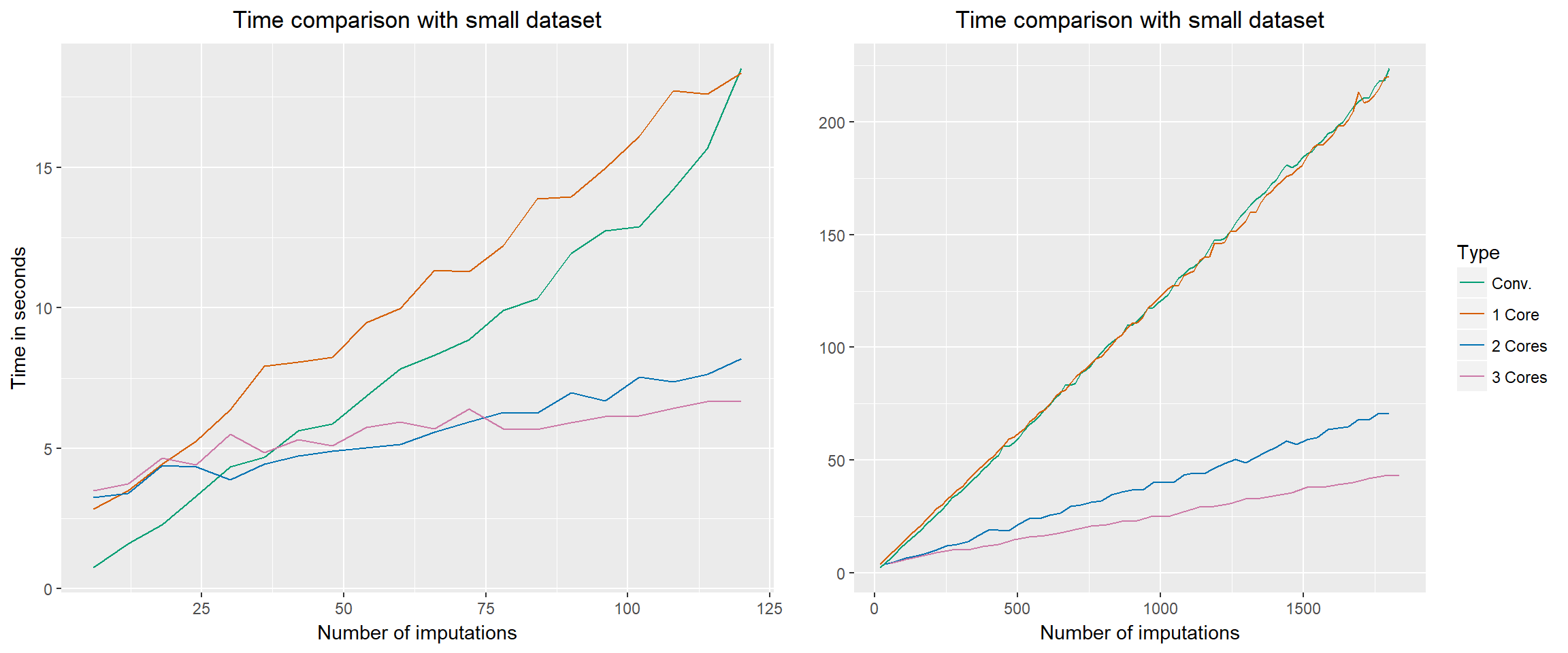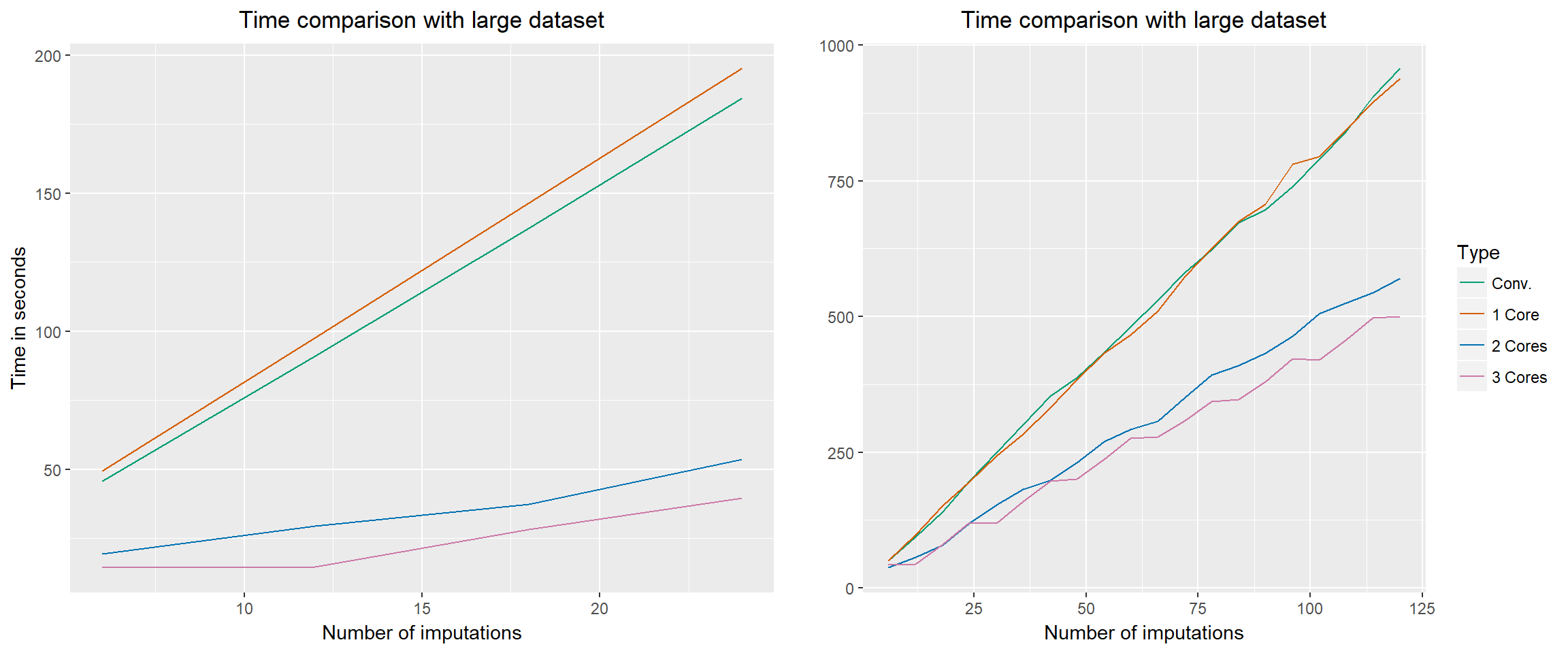For big datasets or high number of imputations, performing multiple imputation with function mice from package mice (Van Buuren & Groothuis-Oudshoorn, 2011) might take a long time. As a solution, wrapper function parlMICE was created to enable the imputation procedure to be run in parallel. This is done by dividing the imputations over multiple cores (or CPUs), thus potentially speeding up the process.

This vignette demonstrates two applications of the parlMICE function. The first application shows the tradeoff between time and increasing number of imputations ($$m$$) for a small; the second application does the same, but for a relatively large dataset. We also discuss parlMICE’s arguments.

The function parlMICE depends on packages parallel and mice. For more information about running functions in parallel, see e.g. the parallel manual or the entry How-to-go parallel in R on Max Gordon’s blog. Function parlMICE found its inspiration from Max’s useful suggestions on parallelization of mice’s chains on stackoverflow.

### Time gain with small datasets

We demonstrate the potential gain in computing efficiency on simulated data. To this end we sample 1,000 cases from a multivariate normal distribution with mean vector

$\mu = \left[\begin{array} {r} 0 \\ 0 \\ 0 \\ 0 \end{array}\right]$

and covariance matrix

$\Sigma = \left[\begin{array} {rrrr} 1&0.5&0.5&0.5 \\ 0.5&1&0.5&0.5 \\ 0.5&0.5&1&0.5 \\ 0.5&0.5&0.5&1 \end{array}\right].$

A MCAR missingness mechanism is imposed on the data where 80 percent of the cases (i.e. rows) has missingness on one variable. All variables have missing values. The missingness is randomly generated with the following arguments from function mice:::ampute:

ampute(data, prop = 0.8)

# EDIT:
# At the time of running these simulations, the defaults in ampute
# resulted in MCAR missingness. The new defaults of ampute
# generate MARRIGHT missingness.

We compare the default ‘sequential’ function mice with function parlMICE. In both functions we use the defaults arguments for the mice algorithm, although these could very easily be changed if desired by the user. To demonstrate the increased efficiency when putting more than one computing core to work, we repeat the procedure with parlMICE for 1, 2 and 3 cores. Figure 1 shows a graphical representation of the results.Figure 1. Processing time for small datasets. Multiple imputations are performed with mice (conventional) and wrapper function parlMICE (1, 2 and 3 cores respectively). The dataset has 1000 cases and 4 variables with a correlation of 0.5. 80 percent of the cases has one missing value based on MCAR missingness.

It becomes apparent that for a small number of imputations, the conventional mice function is faster than the wrapper function parlMICE. This is the case until the number of imputations $$m \leq 35$$. For higher $$m$$, wrapper function parlMICE returns the imputations faster.

The right plot in Figure 1 shows the processing time for very high number of imputations. After the intersection, the lines follow the same trend: 3 cores execute the same amount of work faster than 2 cores, which executes faster than a single core. Naturally, using parlMICE with 1 core is similar to function mice, as the figure shows with the green and orange lines.

### Time gain with large datasets

We replicated the above detailed simulation setup with a larger dataset of 10,000 cases and 8 variables. The mean and covariance structure follow the sampling scheme of the smaller data set. We show the results of this simulation in Figure 2.Figure 2. Processing time for large datasets. Multiple imputations are performed with mice (conventional) and wrapper function parlMICE (1, 2 and 3 cores respectively). The dataset has 10000 cases and 8 variables with a correlation of 0.5. 80 percent of the cases has one missing value based on MCAR missingness.

When datasets are sufficiently large, function parlMICE works faster than mice for all $$m$$. In such cases, even for very small numbers of imputations, running mice in parallel with parlMICE saves a significant amount of time. This gain in efficiency can easily exceed 70 percent for $$m = 10$$ imputations and more.

There is not a large difference between using 2 and 3 cores with wrapper function parlMICE. For all number of imputations, the procedure runs faster with 3 cores, even though the imputations have to be divided over the cores. It might therefore be desirable to use always as many cores as possible, while leaving 1 core out to govern any overhead computing. For example, on a hexacore machine, use only 5 cores to run the mice algorithm in parallel with parlMICE.

### Default settings

We will now discuss the arguments of function parlMICE. Easy imputation of an incomplete dataset (say, nhanes) can be performed with parlMICE in the following way.

imp <- parlMICE(nhanes)
class(imp)
##  "mids"

The function returns a mids object as created by mice. In fact, parlMICE makes use of function ibind to combine the mids objects returned by the different cores. Therefore, the call of the mids object has slightly changed.

imp$call ## [] ## mice(data = data, m = n.imp.core, printFlag = FALSE) ## ## [] ## ibind(x = imp, y = imps[[i]]) ## ## [] ## ibind(x = imp, y = imps[[i]]) All other parts of the mids object are standard. ### Using mice arguments Function parlMICE is able to deal with the conventional mice arguments. In order to change the imputation method from its default (predictive mean matching) to, for example, Bayesian linear regression, the method argument can be adjusted. For other possibilities with mice, we refer to the mice manual. imp <- parlMICE(nhanes, method = "norm") imp$method
##    age    bmi    hyp    chl
## "norm" "norm" "norm" "norm"

In mice, the number of imputations is specified with argument m. In parlMICE, it is possible to use this argument as well, but we advise to rather use the arguments n.core and n.imp.core. The next section discusses these arguments.

### Arguments n.core, n.imp.core

With n.core, the number of cores (or CPUs) is given. n.imp.core defines the number of imputations per core. Subsequently, the total number of imputations equals n.core * n.imp.core.

As a default, n.core is specified as the number of available, logical cores minus 1. The default number of imputations per core has been set to 2. The rational behind this is to on average perform a number of imputations that is close to the default setting in mice, which is $$m = 5$$. On computing machines with 4 available, logical cores, the default number of imputations is $$m = (4 - 1) * 2 = 6$$.

The computer with which this vignette is run, has

detectCores()
##  4

available, logical cores. Consequently, the default parlMICE setting will result to 6 imputations. We can check this by evaluating the $$m$$ that is shown in the mids object.

imp\$m
##  6

### Argument seed

In simulation studies, it is often desired to set a seed to make the results reproducible. In contrast to mice, the seed value for parlMICE cannot be defined outside the function. This has to do with the different cores running at the same time (for more information we gladly refer to the parallel manual).

In parlMICE a seed value can be specified by making use of the argument seed:

imp <- parlMICE(nhanes, seed = 123)

### Systems other than Windows

Function parlMICE calls for function parLapply from the parallel package. Although other options are available, we have chosen for parLapply because it allows for the use of multiple cores on all computers, including a Windows computer. For the cluster, we rely on the default option in the function makeCluster which is "PSOCK".

On systems other than Windows, cluster type "FORK" might be faster (see document How-to-go parallel in R by Max Gordon). Therefore, we advise to change the type to "FORK" if your computer does not run on Windows.

imp <- parlMICE(nhanes, type = "FORK")